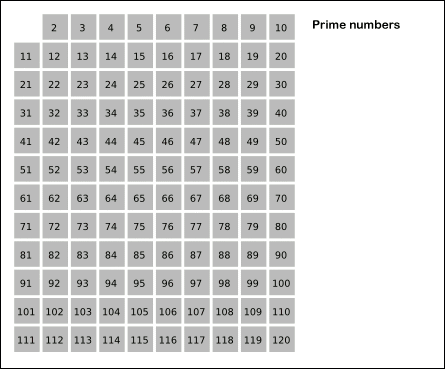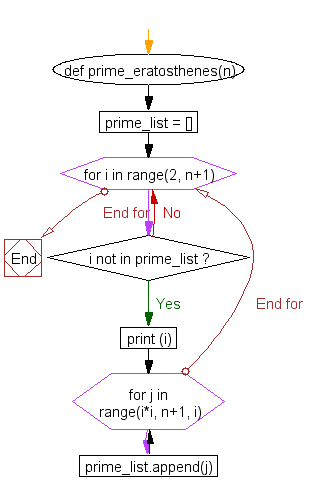﻿ Python: Sieve of Eratosthenes method, for computing prime number - w3resource# Python: Sieve of Eratosthenes method, for computing prime number

## Python List: Exercise - 34 with Solution

Write a Python program using Sieve of Eratosthenes method for computing primes upto a specified number.

Note: In mathematics, the sieve of Eratosthenes (Ancient Greek: κόσκινον Ἐρατοσθένους, kóskinon Eratosthénous), one of a number of prime number sieves, is a simple, ancient algorithm for finding all prime numbers up to any given limit.From Wikipedia Sieve of Eratosthenes: algorithm steps for primes below 121 (including optimization of starting from prime's square).

Sample Solution:-

Python Code:

``````def prime_eratosthenes(n):
prime_list = []
for i in range(2, n+1):
if i not in prime_list:
print (i)
for j in range(i*i, n+1, i):
prime_list.append(j)

print(prime_eratosthenes(100))';
```
```

Sample Output:

```2
3
5
7
11
-------
79
83
89
97
None
```

Flowchart:Python Code Editor:

Have another way to solve this solution? Contribute your code (and comments) through Disqus.

What is the difficulty level of this exercise?

Test your Python skills with w3resource's quiz

﻿

## Python: Tips of the Day

Checks if the given number falls within the given range.

Example:

```def tips_range(n, start, end = 0):
return start <= n <= end if end >= start else end <= n <= start
print(tips_range(2, 4, 6))
print(tips_range(4, 8))
print(tips_range(1, 3, 5))
print(tips_range(1, 3))
```

Output:

```False
True
False
True
```